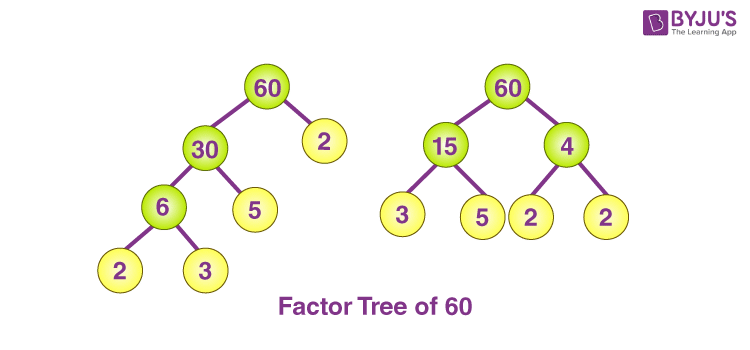# What are two different factor trees for 60?

Since 60 can be expressed as the product of two numbers, its prime factorization remains constant. A composite number’s prime factorization is always unique. We can draw two different factor trees since 60 is a composite number.

The following is the factor trees for 60.

 60 = 2 × 30 30 = 6 × 5 6 = 2 × 3

 60 = 15 × 4 15 = 3 × 5 4 = 2 × 2(15)(5)